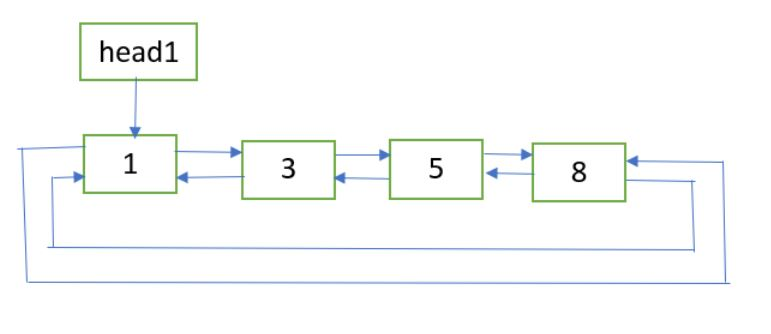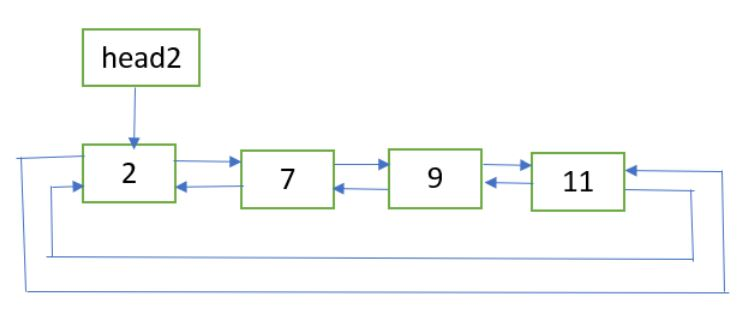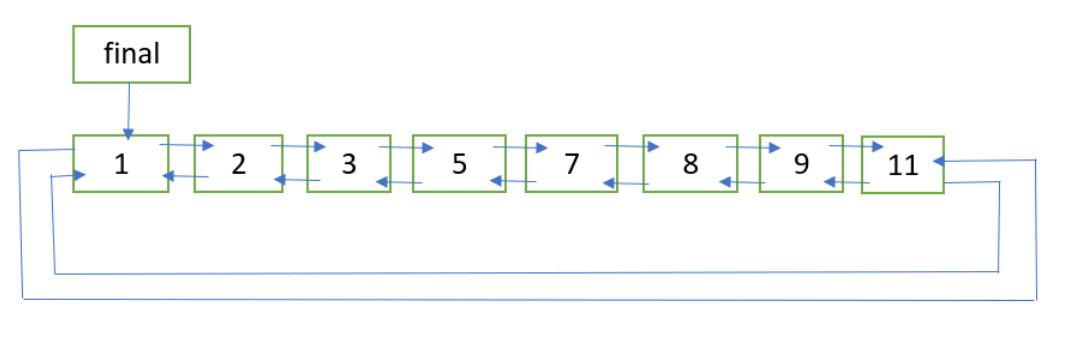# Sorted merge of two sorted doubly circular linked lists

Given two sorted Doubly circular Linked List containing n1 and n2 nodes respectively. The problem is to merge the two lists such that resultant list is also in sorted order.

Example:
List 1:List 2:Final list:## Recommended: Please try your approach on {IDE} first, before moving on to the solution.

Approach: Following are the steps:

3. Let last1 and last2 be the last nodes of the two lists respectively. They can be obtained with the help of the previous links of the first nodes.
4. Get pointer to the node which will be the last node of the final list. If last1.data < last2.data, then last_node = last2, Else last_node = last1.
5. Update last1.next = last2.next = NULL.
6. Now merge the two lists as two sorted doubly linked list are being merged. Refer merge procedure of this post. Let the first node of the final list be finalHead.

## C++

 `// C++ implementation for Sorted merge of two ` `// sorted doubly circular linked list ` `#include ` `using` `namespace` `std; ` ` `  `struct` `Node { ` `    ``int` `data; ` `    ``Node *next, *prev; ` `}; ` ` `  `// A utility function to insert a new node at the ` `// beginning of doubly circular linked list ` `void` `insert(Node** head_ref, ``int` `data) ` `{ ` `    ``// allocate space ` `    ``Node* new_node = ``new` `Node; ` ` `  `    ``// put in the data ` `    ``new_node->data = data; ` ` `  `    ``// if list is empty ` `    ``if` `(*head_ref == NULL) { ` `        ``new_node->next = new_node; ` `        ``new_node->prev = new_node; ` `    ``} ` ` `  `    ``else` `{ ` ` `  `        ``// pointer points to last Node ` `        ``Node* last = (*head_ref)->prev; ` ` `  `        ``// setting up previous and next of new node ` `        ``new_node->next = *head_ref; ` `        ``new_node->prev = last; ` ` `  `        ``// update next and previous pointers of head_ref ` `        ``// and last. ` `        ``last->next = (*head_ref)->prev = new_node; ` `    ``} ` ` `  `    ``// update head_ref pointer ` `    ``*head_ref = new_node; ` `} ` ` `  `// function for Sorted merge of two ` `// sorted doubly linked list ` `Node* merge(Node* first, Node* second) ` `{ ` `    ``// If first list is empty ` `    ``if` `(!first) ` `        ``return` `second; ` ` `  `    ``// If second list is empty ` `    ``if` `(!second) ` `        ``return` `first; ` ` `  `    ``// Pick the smaller value and adjust ` `    ``// the links ` `    ``if` `(first->data < second->data) { ` `        ``first->next = merge(first->next, second); ` `        ``first->next->prev = first; ` `        ``first->prev = NULL; ` `        ``return` `first; ` `    ``} ` `    ``else` `{ ` `        ``second->next = merge(first, second->next); ` `        ``second->next->prev = second; ` `        ``second->prev = NULL; ` `        ``return` `second; ` `    ``} ` `} ` ` `  `// function for Sorted merge of two sorted ` `// doubly circular linked list ` `Node* mergeUtil(Node* head1, Node* head2) ` `{ ` `    ``// if 1st list is empty ` `    ``if` `(!head1) ` `        ``return` `head2; ` ` `  `    ``// if 2nd list is empty ` `    ``if` `(!head2) ` `        ``return` `head1; ` ` `  `    ``// get pointer to the node which will be the ` `    ``// last node of the final list ` `    ``Node* last_node; ` `    ``if` `(head1->prev->data < head2->prev->data) ` `        ``last_node = head2->prev; ` `    ``else` `        ``last_node = head1->prev; ` ` `  `    ``// store NULL to the 'next' link of the last nodes ` `    ``// of the two lists ` `    ``head1->prev->next = head2->prev->next = NULL; ` ` `  `    ``// sorted merge of head1 and head2 ` `    ``Node* finalHead = merge(head1, head2); ` ` `  `    ``// 'prev' of 1st node pointing the last node ` `    ``// 'next' of last node pointing to 1st node ` `    ``finalHead->prev = last_node; ` `    ``last_node->next = finalHead; ` ` `  `    ``return` `finalHead; ` `} ` ` `  `// function to print the list ` `void` `printList(Node* head) ` `{ ` `    ``Node* temp = head; ` ` `  `    ``while` `(temp->next != head) { ` `        ``cout << temp->data << ``" "``; ` `        ``temp = temp->next; ` `    ``} ` `    ``cout << temp->data << ``" "``; ` `} ` ` `  `// Driver program to test above ` `int` `main() ` `{ ` `    ``Node *head1 = NULL, *head2 = NULL; ` ` `  `    ``// list 1: ` `    ``insert(&head1, 8); ` `    ``insert(&head1, 5); ` `    ``insert(&head1, 3); ` `    ``insert(&head1, 1); ` ` `  `    ``// list 2: ` `    ``insert(&head2, 11); ` `    ``insert(&head2, 9); ` `    ``insert(&head2, 7); ` `    ``insert(&head2, 2); ` ` `  `    ``Node* newHead = mergeUtil(head1, head2); ` ` `  `    ``cout << ``"Final Sorted List: "``; ` `    ``printList(newHead); ` ` `  `    ``return` `0; ` `} `

## Java

 `// Java implementation for Sorted merge of two  ` `// sorted doubly circular linked list  ` `class` `GFG ` `{ ` `     `  `static` `class` `Node ` `{  ` `    ``int` `data;  ` `    ``Node next, prev;  ` `};  ` ` `  `// A utility function to insert a new node at the  ` `// beginning of doubly circular linked list  ` `static` `Node insert(Node head_ref, ``int` `data)  ` `{  ` `    ``// allocate space  ` `    ``Node new_node = ``new` `Node();  ` ` `  `    ``// put in the data  ` `    ``new_node.data = data;  ` ` `  `    ``// if list is empty  ` `    ``if` `(head_ref == ``null``) ` `    ``{  ` `        ``new_node.next = new_node;  ` `        ``new_node.prev = new_node;  ` `    ``}  ` ` `  `    ``else` `    ``{  ` ` `  `        ``// pointer points to last Node  ` `        ``Node last = (head_ref).prev;  ` ` `  `        ``// setting up previous and next of new node  ` `        ``new_node.next = head_ref;  ` `        ``new_node.prev = last;  ` ` `  `        ``// update next and previous pointers of head_ref  ` `        ``// and last.  ` `        ``last.next = (head_ref).prev = new_node;  ` `    ``}  ` ` `  `    ``// update head_ref pointer  ` `    ``head_ref = new_node;  ` `    ``return` `head_ref; ` `}  ` ` `  `// function for Sorted merge of two  ` `// sorted doubly linked list  ` `static` `Node merge(Node first, Node second)  ` `{  ` `    ``// If first list is empty  ` `    ``if` `(first == ``null``)  ` `        ``return` `second;  ` ` `  `    ``// If second list is empty  ` `    ``if` `(second == ``null``)  ` `        ``return` `first;  ` ` `  `    ``// Pick the smaller value and adjust  ` `    ``// the links  ` `    ``if` `(first.data < second.data)  ` `    ``{  ` `        ``first.next = merge(first.next, second);  ` `        ``first.next.prev = first;  ` `        ``first.prev = ``null``;  ` `        ``return` `first;  ` `    ``}  ` `    ``else`  `    ``{  ` `        ``second.next = merge(first, second.next);  ` `        ``second.next.prev = second;  ` `        ``second.prev = ``null``;  ` `        ``return` `second;  ` `    ``}  ` `}  ` ` `  `// function for Sorted merge of two sorted  ` `// doubly circular linked list  ` `static` `Node mergeUtil(Node head1, Node head2)  ` `{  ` `    ``// if 1st list is empty  ` `    ``if` `(head1 == ``null``)  ` `        ``return` `head2;  ` ` `  `    ``// if 2nd list is empty  ` `    ``if` `(head2 == ``null``)  ` `        ``return` `head1;  ` ` `  `    ``// get pointer to the node which will be the  ` `    ``// last node of the final list  ` `    ``Node last_node;  ` `    ``if` `(head1.prev.data < head2.prev.data)  ` `        ``last_node = head2.prev;  ` `    ``else` `        ``last_node = head1.prev;  ` ` `  `    ``// store null to the 'next' link of the last nodes  ` `    ``// of the two lists  ` `    ``head1.prev.next = head2.prev.next = ``null``;  ` ` `  `    ``// sorted merge of head1 and head2  ` `    ``Node finalHead = merge(head1, head2);  ` ` `  `    ``// 'prev' of 1st node pointing the last node  ` `    ``// 'next' of last node pointing to 1st node  ` `    ``finalHead.prev = last_node;  ` `    ``last_node.next = finalHead;  ` ` `  `    ``return` `finalHead;  ` `}  ` ` `  `// function to print the list  ` `static` `void` `printList(Node head)  ` `{  ` `    ``Node temp = head;  ` ` `  `    ``while` `(temp.next != head) ` `    ``{  ` `        ``System.out.print ( temp.data+ ``" "``);  ` `        ``temp = temp.next;  ` `    ``}  ` `    ``System.out.print ( temp.data + ``" "``);  ` `}  ` ` `  `// Driver code  ` `public` `static` `void` `main(String args[]) ` `{  ` `    ``Node head1 = ``null``, head2 = ``null``;  ` ` `  `    ``// list 1:  ` `    ``head1 = insert(head1, ``8``);  ` `    ``head1 = insert(head1, ``5``);  ` `    ``head1 = insert(head1, ``3``);  ` `    ``head1 = insert(head1, ``1``);  ` ` `  `    ``// list 2:  ` `    ``head2 = insert(head2, ``11``);  ` `    ``head2 = insert(head2, ``9``);  ` `    ``head2 = insert(head2, ``7``);  ` `    ``head2 = insert(head2, ``2``);  ` ` `  `    ``Node newHead = mergeUtil(head1, head2);  ` ` `  `    ``System.out.print( ``"Final Sorted List: "``);  ` `    ``printList(newHead);  ` `} ` `}  ` ` `  `// This code is contributed by Arnab Kundu `

## Python3

 `    ``# Python3 implementation for Sorted merge  ` `# of two sorted doubly circular linked list ` `import` `math ` ` `  `class` `Node:  ` `    ``def` `__init__(``self``, data):  ` `        ``self``.data ``=` `data  ` `        ``self``.``next` `=` `None` `        ``self``.prev ``=` `None` ` `  `# A utility function to insert ` `# a new node at the beginning  ` `# of doubly circular linked list ` `def` `insert(head_ref, data): ` `     `  `    ``# allocate space ` `    ``new_node ``=` `Node(data) ` ` `  `    ``# put in the data ` `    ``new_node.data ``=` `data ` ` `  `    ``# if list is empty ` `    ``if` `(head_ref ``=``=` `None``): ` `        ``new_node.``next` `=` `new_node ` `        ``new_node.prev ``=` `new_node ` `     `  `    ``else` `: ` ` `  `        ``# pointer points to last Node ` `        ``last ``=` `head_ref.prev ` ` `  `        ``# setting up previous and  ` `        ``# next of new node ` `        ``new_node.``next` `=` `head_ref ` `        ``new_node.prev ``=` `last ` ` `  `        ``# update next and previous pointers  ` `        ``# of head_ref and last. ` `        ``last.``next` `=` `new_node ` `        ``head_ref.prev ``=` `new_node ` `     `  `    ``# update head_ref pointer ` `    ``head_ref ``=` `new_node ` `    ``return` `head_ref ` ` `  `# function for Sorted merge of two ` `# sorted doubly linked list ` `def` `merge(first, second): ` `     `  `    ``# If first list is empty ` `    ``if` `(first ``=``=` `None``): ` `        ``return` `second ` ` `  `    ``# If second list is empty ` `    ``if` `(second ``=``=` `None``): ` `        ``return` `first ` ` `  `    ``# Pick the smaller value and  ` `    ``# adjust the links ` `    ``if` `(first.data < second.data) : ` `        ``first.``next` `=` `merge(first.``next``,  ` `                           ``second) ` `        ``first.``next``.prev ``=` `first ` `        ``first.prev ``=` `None` `        ``return` `first ` `     `  `    ``else` `: ` `        ``second.``next` `=` `merge(first,  ` `                            ``second.``next``) ` `        ``second.``next``.prev ``=` `second ` `        ``second.prev ``=` `None` `        ``return` `second ` `     `  `# function for Sorted merge of two sorted ` `# doubly circular linked list ` `def` `mergeUtil(head1, head2): ` `     `  `    ``# if 1st list is empty ` `    ``if` `(head1 ``=``=` `None``): ` `        ``return` `head2 ` ` `  `    ``# if 2nd list is empty ` `    ``if` `(head2 ``=``=` `None``): ` `        ``return` `head1 ` ` `  `    ``# get pointer to the node  ` `    ``# which will be the last node  ` `    ``# of the final list last_node ` `    ``if` `(head1.prev.data < head2.prev.data): ` `        ``last_node ``=` `head2.prev ` `    ``else``: ` `        ``last_node ``=` `head1.prev ` ` `  `    ``# store None to the 'next' link of ` `    ``# the last nodes of the two lists ` `    ``head1.prev.``next` `=` `None` `    ``head2.prev.``next` `=` `None` ` `  `    ``# sorted merge of head1 and head2 ` `    ``finalHead ``=` `merge(head1, head2) ` ` `  `    ``# 'prev' of 1st node pointing the last node ` `    ``# 'next' of last node pointing to 1st node ` `    ``finalHead.prev ``=` `last_node ` `    ``last_node.``next` `=` `finalHead ` ` `  `    ``return` `finalHead ` ` `  `# function to print the list ` `def` `printList(head): ` `    ``temp ``=` `head ` ` `  `    ``while` `(temp.``next` `!``=` `head): ` `        ``print``(temp.data, end ``=` `" "``) ` `        ``temp ``=` `temp.``next` `     `  `    ``print``(temp.data, end ``=` `" "``) ` ` `  `# Driver Code ` `if` `__name__``=``=``'__main__'``:  ` `    ``head1 ``=` `None` `    ``head2 ``=` `None` ` `  `    ``# list 1: ` `    ``head1 ``=` `insert(head1, ``8``) ` `    ``head1 ``=` `insert(head1, ``5``) ` `    ``head1 ``=` `insert(head1, ``3``) ` `    ``head1 ``=` `insert(head1, ``1``) ` ` `  `    ``# list 2: ` `    ``head2 ``=` `insert(head2, ``11``) ` `    ``head2 ``=` `insert(head2, ``9``) ` `    ``head2 ``=` `insert(head2, ``7``) ` `    ``head2 ``=` `insert(head2, ``2``) ` ` `  `    ``newHead ``=` `mergeUtil(head1, head2) ` ` `  `    ``print``(``"Final Sorted List: "``, end ``=` `"") ` `    ``printList(newHead) ` ` `  `# This code is contributed by Srathore `

## C#

 `// C# implementation for Sorted merge of two  ` `// sorted doubly circular linked list  ` `using` `System; ` ` `  `class` `GFG ` `{ ` `     `  `public` `class` `Node ` `{  ` `    ``public` `int` `data;  ` `    ``public` `Node next, prev;  ` `};  ` ` `  `// A utility function to insert a new node at the  ` `// beginning of doubly circular linked list  ` `static` `Node insert(Node head_ref, ``int` `data)  ` `{  ` `    ``// allocate space  ` `    ``Node new_node = ``new` `Node();  ` ` `  `    ``// put in the data  ` `    ``new_node.data = data;  ` ` `  `    ``// if list is empty  ` `    ``if` `(head_ref == ``null``) ` `    ``{  ` `        ``new_node.next = new_node;  ` `        ``new_node.prev = new_node;  ` `    ``}  ` ` `  `    ``else` `    ``{  ` ` `  `        ``// pointer points to last Node  ` `        ``Node last = (head_ref).prev;  ` ` `  `        ``// setting up previous and next of new node  ` `        ``new_node.next = head_ref;  ` `        ``new_node.prev = last;  ` ` `  `        ``// update next and previous pointers of head_ref  ` `        ``// and last.  ` `        ``last.next = (head_ref).prev = new_node;  ` `    ``}  ` ` `  `    ``// update head_ref pointer  ` `    ``head_ref = new_node;  ` `    ``return` `head_ref; ` `}  ` ` `  `// function for Sorted merge of two  ` `// sorted doubly linked list  ` `static` `Node merge(Node first, Node second)  ` `{  ` `    ``// If first list is empty  ` `    ``if` `(first == ``null``)  ` `        ``return` `second;  ` ` `  `    ``// If second list is empty  ` `    ``if` `(second == ``null``)  ` `        ``return` `first;  ` ` `  `    ``// Pick the smaller value and adjust  ` `    ``// the links  ` `    ``if` `(first.data < second.data)  ` `    ``{  ` `        ``first.next = merge(first.next, second);  ` `        ``first.next.prev = first;  ` `        ``first.prev = ``null``;  ` `        ``return` `first;  ` `    ``}  ` `    ``else` `    ``{  ` `        ``second.next = merge(first, second.next);  ` `        ``second.next.prev = second;  ` `        ``second.prev = ``null``;  ` `        ``return` `second;  ` `    ``}  ` `}  ` ` `  `// function for Sorted merge of two sorted  ` `// doubly circular linked list  ` `static` `Node mergeUtil(Node head1, Node head2)  ` `{  ` `    ``// if 1st list is empty  ` `    ``if` `(head1 == ``null``)  ` `        ``return` `head2;  ` ` `  `    ``// if 2nd list is empty  ` `    ``if` `(head2 == ``null``)  ` `        ``return` `head1;  ` ` `  `    ``// get pointer to the node which will be the  ` `    ``// last node of the final list  ` `    ``Node last_node;  ` `    ``if` `(head1.prev.data < head2.prev.data)  ` `        ``last_node = head2.prev;  ` `    ``else` `        ``last_node = head1.prev;  ` ` `  `    ``// store null to the 'next' link of the last nodes  ` `    ``// of the two lists  ` `    ``head1.prev.next = head2.prev.next = ``null``;  ` ` `  `    ``// sorted merge of head1 and head2  ` `    ``Node finalHead = merge(head1, head2);  ` ` `  `    ``// 'prev' of 1st node pointing the last node  ` `    ``// 'next' of last node pointing to 1st node  ` `    ``finalHead.prev = last_node;  ` `    ``last_node.next = finalHead;  ` ` `  `    ``return` `finalHead;  ` `}  ` ` `  `// function to print the list  ` `static` `void` `printList(Node head)  ` `{  ` `    ``Node temp = head;  ` ` `  `    ``while` `(temp.next != head) ` `    ``{  ` `        ``Console.Write(temp.data + ``" "``);  ` `        ``temp = temp.next;  ` `    ``}  ` `    ``Console.Write(temp.data + ``" "``);  ` `}  ` ` `  `// Driver code  ` `public` `static` `void` `Main() ` `{  ` `    ``Node head1 = ``null``, head2 = ``null``;  ` ` `  `    ``// list 1:  ` `    ``head1 = insert(head1, 8);  ` `    ``head1 = insert(head1, 5);  ` `    ``head1 = insert(head1, 3);  ` `    ``head1 = insert(head1, 1);  ` ` `  `    ``// list 2:  ` `    ``head2 = insert(head2, 11);  ` `    ``head2 = insert(head2, 9);  ` `    ``head2 = insert(head2, 7);  ` `    ``head2 = insert(head2, 2);  ` ` `  `    ``Node newHead = mergeUtil(head1, head2);  ` ` `  `    ``Console.Write( ``"Final Sorted List: "``);  ` `    ``printList(newHead);  ` `} ` `}  ` ` `  `// This code is contributed by Princi Singh `

Output:

```Final Sorted List: 1 2 3 5 7 8 9 11
```

Time Complexity: O(n1 + n2).

Attention reader! Don’t stop learning now. Get hold of all the important DSA concepts with the DSA Self Paced Course at a student-friendly price and become industry ready.

My Personal Notes arrow_drop_upCheck out this Author's contributed articles.

If you like GeeksforGeeks and would like to contribute, you can also write an article using contribute.geeksforgeeks.org or mail your article to contribute@geeksforgeeks.org. See your article appearing on the GeeksforGeeks main page and help other Geeks.

Please Improve this article if you find anything incorrect by clicking on the "Improve Article" button below.

Article Tags :
Practice Tags :

2

Please write to us at contribute@geeksforgeeks.org to report any issue with the above content.